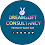### System Verilog (Part C) - Language Constructs II• Arrays in System Verilog: An array in any programming language is a system variable which has a fixed length, and it cannot be changed after it has been created.
• It holds a fixed number of equally sized data types.
• While declaring an array, you can access the individual elements of array by using the index.
• Fixed/Static arrays:
• Packed arrays: When you put the declaration on the left side, it is a packed array.
• Stores continuously in the memory.
Example: bit [7:0] a; //packed array of 8 bits
• Unpacked arrays: When you put the declaration on the left side, it is an unpacked array
• No guarantee that it will store the data continuously in the memory.
Example: bit a[7:0]; //unpacked array of 8 bits
• Multidimensional arrays: You can declare as packed or unpacked, or as a combination of packed and unpacked.
Example 1: bit [3:0][7:0] a; /* 2D packed array of 8 bit or byte length and there are 4 bytes in this array */

Example 2: bit a [3:0][7:0]; /* 2D Unpacked array */

Example 3: bit [7:0] a; /* Unpacked array of 3 bytes. Each byte is packed. */

bit [7:0] a_unpacked;

a_array 
 7 6 5 4 3 2 1 0
a_array 
 7 6 5 4 3 2 1 0
a_array 
 7 6 5 4 3 2 1 0

• System Verilog supports Dynamic Arrays, Associative Arrays and Queues: Only used for verification and not for synthesizable RTL code.

• Operators:
• Arithmetic
 -a Negate a+b Add a-b Subtract a*b Multiply a/b Divide a%b Modulus a**b Exponentiate a<>b Logical right shift a<<>>b Arithmetic right shift
•   Logical

 !a NOT a&&b AND a||b OR a==b a!=b [in]equality Returns x when x or z are in bits. Else returns 0 or 1. a===b a!==b bitwise equality, i.e. equality will pass only if they are maching bit-by-bit. Therefore, it will take care of x and z values also. case [in] equality returns 0 or 1 based on bit by bit comparison.

• Relational

 a>b greater than a>=b greater than or equal a

• Bitwise

 ~a NOT a&b AND a|b OR a^b XOR a~^b a^~b XNOR

• Reduction: e.g. individual bits of 'a' will perform AND logic together

 &a AND ~&a NAND |a OR ~|a NOR ^a XOR ~^a ^~a XNOR

• Conditional

 a?b:c If a then b else c

• => If the condition is TRUE, then expression b is evaluated, if condition is FALSE then expression c is evaluated.
Example: assign result= (a==b) ? 1: 0;
=> if condition is TRUE, 1 is returned by the operator and if it is not TRUE i.e. the value of a and b are not equal then 0 is returned by the operator and assigned to the 'result'.

1.